# Third grade Math – Subtraction Apk by Sergey Malugin

#### Gallery: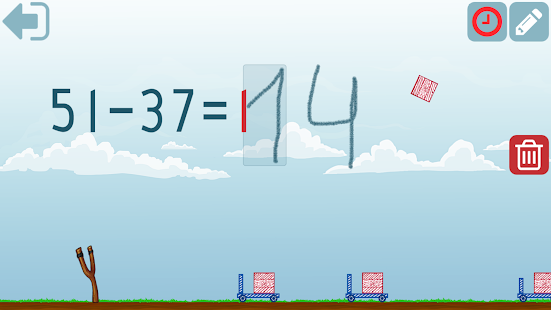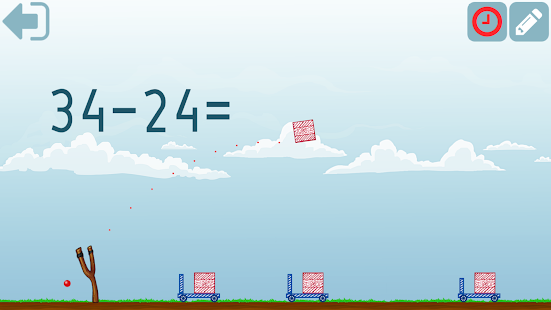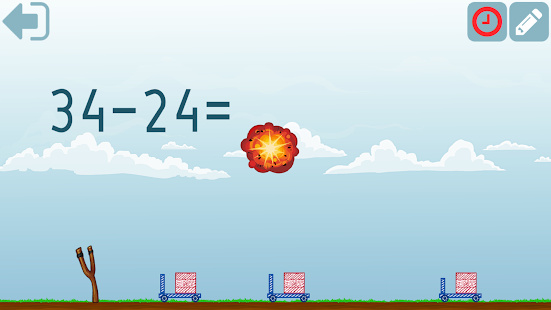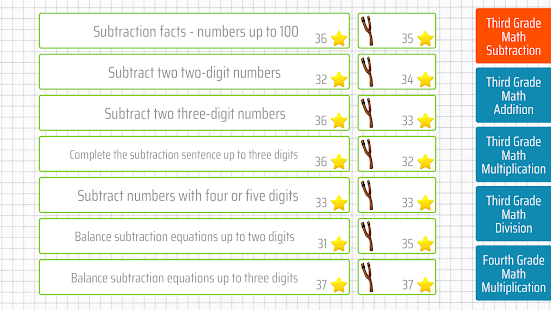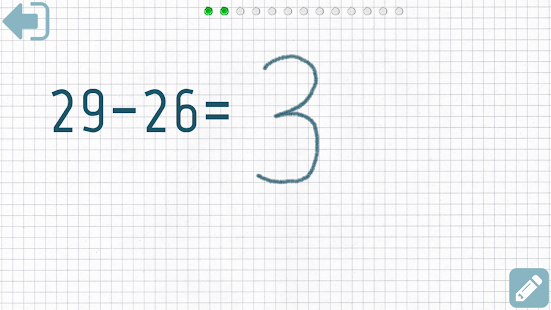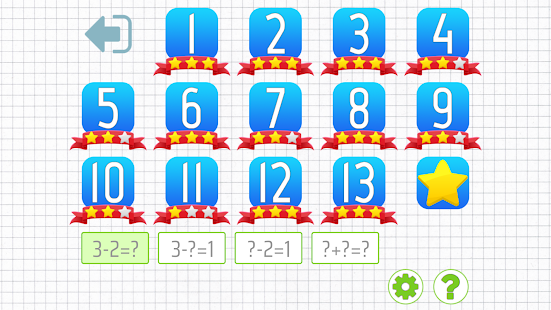#### About Third grade Math – Subtraction

Intuitive interface powered by handwriting input and a fun and engaging mini game in addition to a regular math trainer mode make our app stand out from the crowd of generic math learning apps.

With Third grade Math – Subtraction you can practice and improve the following math skills:
– Subtraction facts – numbers up to 100
– Subtract two two-digit numbers
– Subtract two three-digit numbers
– Complete the subtraction sentence up to three digits
– Subtract numbers with four or five digits
– Balance subtraction equations up to two digits
– Balance subtraction equations up to three digits

Choose between two different modes:
– Math Trainer
– Math Shot mini-game

Math Trainer mode helps you to master the math facts, you can solve math problems at you own pace without any time constrain.

Math Shot is a math mini-game with fun and engaging game-play and as we all know learning through play and fun is more effective. The game difficulty adapts to player's skills and makes the game suitable for all ages. Math Shot helps students to develop math fact fluency – the result of consistent learning, repetition, and practice.

#### Third grade Math – Subtraction APK details:

• App Name: Third grade Math – Subtraction
• Current Version: 2.0.0
• Price: Check in PlayStore
• Updated: September 29, 2017
• Content Rating: Everyone
• Android Version: 4.0 and up